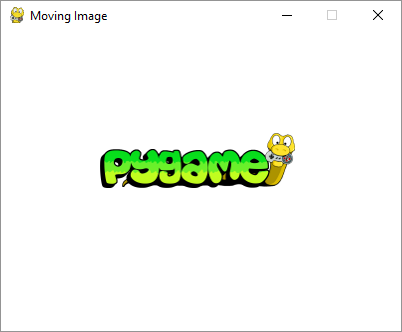# Pygame - Moving an Image

Movement of an object is an important aspect of any computer game. A computer game creates illusion of movement by drawing and erasing an object at incremental position. Following code draws an image by incrementing x coordinate position in an event loop and erasing it with the background color.

## Example

image_filename = 'pygame.png'
import pygame
from pygame.locals import *
from sys import exit
pygame.init()
screen = pygame.display.set_mode((400,300), 0, 32)
pygame.display.set_caption("Moving Image")
x = 0
while True:
screen.fill((255,255,255))
for event in pygame.event.get():
if event.type == QUIT:
exit()
screen.blit(img, (x, 100))
x= x+0.5

if x > 400:
x = x-400
pygame.display.update()


## Output

The Pygame logo image starts displaying at left border and repeatedly shifts towards right. If it reaches right border, its position is reset to left.In the following program, the image is displayed at (0,150) position to begin with. When user presses arrow keys (left, right, up, down), the image changes its location by 5 pixels. If a KEYDOWN event occurs, the program checks if the key value is K_LEFT, K_RIGHT, K_UP or K_DOWN. The x coordinate changes by +5 or -5 if it is K_LEFT or K_RIGHT. Value of y coordinate changes by -5 or +5 if key value is K_UP or K_DOWN.

image_filename = 'pygame.png'
import pygame
from pygame.locals import *
from sys import exit
pygame.init()
screen = pygame.display.set_mode((400,300))
pygame.display.set_caption("Moving with arrows")
x = 0
y= 150
while True:
screen.fill((255,255,255))
screen.blit(img, (x, y))
for event in pygame.event.get():
if event.type == QUIT:
exit()

if event.type == KEYDOWN:
if event.key == K_RIGHT:
x= x+5
if event.key == K_LEFT:
x=x-5
if event.key == K_UP:
y=y-5
if event.key == K_DOWN:
y=y+5
pygame.display.update()